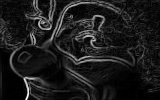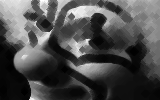# ImageJ Ops¶

ImageJ Ops is a library for N-dimensional image processing. The primary design goals of Ops are:

1. Ease of use. Ops provides a wealth of easy-to-use image processing operations ("ops").
2. Reusability. Ops extends Java's mantra of "write once, run anywhere" to image processing algorithms. Algorithms written in the Ops framework are usable as-is from any SciJava-compatible software project, such as ImageJ, CellProfiler, KNIME, OMERO and Alida.
3. Reproducibility. Ops are deterministic: calling the same op twice with the same arguments yields the same result, always. And all ops are versioned.
4. Power. An op may consist of any number of typed input and output parameters. Ops may operate on arbitrary data structures, including images of N dimensions stored in a myriad of different ways: as files on disk, programmatically generated in memory, or in remote databases.
5. Extensibility. Ops provides a robust framework for writing new ops, and for extending existing ops in new directions. See the "Extending ImageJ - Ops" tutorial notebook for details.
6. Speed. The Ops framework provides a means to override any general-but-slow op with a faster-but-more-specific alternative, fully transparently to the user.

## Quick Start Guide¶

### Getting started¶

In :
%classpath config resolver scijava.public https://maven.scijava.org/content/groups/public
%classpath add mvn net.imagej imagej 2.0.0-rc-71
ij = new net.imagej.ImageJ()
"ImageJ ${ij.getVersion()} is ready to go."  Added new repo: scijava.public  Out: ImageJ 2.0.0-rc-71 is ready to go. Let's open up the friendly Clown image to use for our experiments. (For the coulrophobic, feel free to use the Fluorescent Cells instead.) In : clown = ij.io().open("https://imagej.net/images/clown.png")  Out:It's a bit large, so let's scale it down. We'll use the transform.scaleView op. For succinctness, we'll write only scaleView rather than the fully qualified op name transform.scaleView. We'll use N-linear interpolation, since it tends to look pretty nice. In : import net.imglib2.interpolation.randomaccess.NLinearInterpolatorFactory scaleFactors = [0.5, 0.5, 1] // Reduce X and Y to 50%; leave C dimension alone. interpolationStrategy = new NLinearInterpolatorFactory() image = ij.op().run("scaleView", clown, scaleFactors, interpolationStrategy)  Out:Let's also make a single-channel 32-bit floating point version: In : import net.imglib2.FinalInterval w = image.dimension(0); h = image.dimension(1) slice = FinalInterval.createMinSize(0, 0, 0, w, h, 1) grayImage = ij.op().run("crop", image, slice, true) image32 = ij.op().convert().float32(grayImage)  Out:Finally, let's define a function for showing multiple uniformly scaled images: In : import net.imglib2.RandomAccessibleInterval tile = { images -> int[] gridLayout = images in List ? [images.size, images.size] : // 2D images list [images.size] // 1D images list RandomAccessibleInterval[] rais = images.flatten() ij.notebook().mosaic(gridLayout, rais) }  Out: [email protected] ### Learning about available ops¶ You can get a complete list of available ops using the ops() method. But it is a bit overwhelming, so here is a structured version organized by namespace: In : import net.imagej.ops.OpUtils opsByNS = [:] ij.op().ops().each{op -> ns = OpUtils.getNamespace(op) name = OpUtils.stripNamespace(op) if (!opsByNS.containsKey(ns)) opsByNS.put(ns, name) else opsByNS.put(ns, opsByNS.get(ns) + ', ' + name) } opsByNS.put('<global>', opsByNS.remove(null)) ij.notebook().display(opsByNS)  Out:  coloc icq, kendallTau, maxTKendallTau, pValue, pearsons convert bit, cfloat32, cfloat64, clip, copy, float32, float64, imageType, int16, int32, int64, int8, normalizeScale, scale, uint12, uint128, uint16, uint2, uint32, uint4, uint64, uint8 copy img, imgLabeling, iterableInterval, labelingMapping, rai, type create img, imgFactory, imgLabeling, imgPlus, integerType, kernel, kernel2ndDerivBiGauss, kernelBiGauss, kernelDiffraction, kernelGabor, kernelGaborComplexDouble, kernelGaborDouble, kernelGauss, kernelLog, kernelSobel, labelingMapping, nativeType, object deconvolve accelerate, firstGuess, normalizationFactor, richardsonLucy, richardsonLucyCorrection, richardsonLucyTV, richardsonLucyUpdate filter addNoise, addPoissonNoise, allPartialDerivatives, bilateral, convolve, correlate, createFFTOutput, derivativeGauss, dog, fft, fftSize, frangiVesselness, gauss, hessian, ifft, linearFilter, max, mean, median, min, padFFTInput, padInput, padShiftFFTKernel, paddingIntervalCentered, paddingIntervalOrigin, partialDerivative, sigma, sobel, tubeness, variance geom boundarySize, boundarySizeConvexHull, boundingBox, boxivity, centerOfGravity, centroid, circularity, compactness, contour, convexHull, convexity, eccentricity, feretsAngle, feretsDiameter, mainElongation, majorAxis, marchingCubes, maximumFeret, maximumFeretsAngle, maximumFeretsDiameter, medianElongation, minimumFeret, minimumFeretsAngle, minimumFeretsDiameter, minorAxis, roundness, secondMoment, size, sizeConvexHull, smallestEnclosingBoundingBox, solidity, spareness, sphericity, vertexInterpolator, verticesCount, verticesCountConvexHull, voxelization haralick asm, clusterPromenence, clusterShade, contrast, correlation, differenceEntropy, differenceVariance, entropy, icm1, icm2, ifdm, maxProbability, sumAverage, sumEntropy, sumVariance, textureHomogeneity, variance hog hog image ascii, cooccurrenceMatrix, distancetransform, equation, fill, histogram, integral, invert, normalize, squareIntegral, watershed imagemoments centralMoment00, centralMoment01, centralMoment02, centralMoment03, centralMoment10, centralMoment11, centralMoment12, centralMoment20, centralMoment21, centralMoment30, huMoment1, huMoment2, huMoment3, huMoment4, huMoment5, huMoment6, huMoment7, moment00, moment01, moment10, moment11, normalizedCentralMoment02, normalizedCentralMoment03, normalizedCentralMoment11, normalizedCentralMoment12, normalizedCentralMoment20, normalizedCentralMoment21, normalizedCentralMoment30 labeling cca, merge lbp lbp2D linalg rotate logic and, conditional, equal, greaterThan, greaterThanOrEqual, lessThan, lessThanOrEqual, not, notEqual, or, xor math abs, add, and, arccos, arccosh, arccot, arccoth, arccsc, arccsch, arcsec, arcsech, arcsin, arcsinh, arctan, arctanh, assign, ceil, complement, complexConjugateMultiply, cos, cosh, cot, coth, csc, csch, cubeRoot, divide, exp, expMinusOne, floor, gamma, invert, leftShift, log, log10, log2, logOnePlusX, max, min, multiply, nearestInt, negate, or, power, randomGaussian, randomUniform, reciprocal, remainder, rightShift, round, sec, sech, signum, sin, sinc, sincPi, sinh, sqr, sqrt, step, subtract, tan, tanh, ulp, unsignedRightShift, xor, zero morphology blackTopHat, close, dilate, erode, extractHoles, fillHoles, floodFill, open, outline, thinGuoHall, thinHilditch, thinMorphological, thinZhangSuen, topHat segment detectJunctions, detectRidges stats geometricMean, harmonicMean, integralMean, integralSum, integralVariance, kurtosis, leastSquares, max, mean, median, min, minMax, moment1AboutMean, moment2AboutMean, moment3AboutMean, moment4AboutMean, percentile, quantile, size, skewness, stdDev, sum, sumOfInverses, sumOfLogs, sumOfSquares, variance tamura coarseness, contrast, directionality thread chunker threshold apply, huang, ij1, intermodes, isoData, li, localBernsenThreshold, localContrastThreshold, localMeanThreshold, localMedianThreshold, localMidGreyThreshold, localNiblackThreshold, localPhansalkarThreshold, localSauvolaThreshold, maxEntropy, maxLikelihood, mean, minError, minimum, moments, otsu, percentile, renyiEntropy, rosin, shanbhag, triangle, yen topology boxCount, eulerCharacteristic26N, eulerCharacteristic26NFloating, eulerCorrection transform addDimensionView, collapseNumericView, collapseRealView, collapseView, concatenateView, crop, dropSingletonDimensionsView, extendBorderView, extendMirrorDoubleView, extendMirrorSingleView, extendPeriodicView, extendRandomView, extendValueView, extendView, extendZeroView, flatIterableView, hyperSliceView, interpolateView, intervalView, invertAxisView, offsetView, permuteCoordinatesInverseView, permuteCoordinatesView, permuteView, project, rasterView, rotateView, scaleView, shearView, stackView, subsampleView, translateView, unshearView, zeroMinView zernike magnitude, phase eval, help, identity, join, loop, map, op, run, slice There is a helpful help op which prints out information about an op. We can use it to better understand what sorts of operations are possible, and what kinds of arguments they expect: In : ij.op().help('gauss')  Out: Available operations: (RandomAccessibleInterval out) = net.imagej.ops.filter.gauss.DefaultGaussRA( RandomAccessibleInterval out, RandomAccessible in, double[] sigmas) (RandomAccessibleInterval out?) = net.imagej.ops.filter.gauss.GaussRAISingleSigma( RandomAccessibleInterval out?, RandomAccessibleInterval in, double sigma, OutOfBoundsFactory outOfBounds?) (RandomAccessibleInterval out?) = net.imagej.ops.filter.gauss.DefaultGaussRAI( RandomAccessibleInterval out?, RandomAccessibleInterval in, double[] sigmas, OutOfBoundsFactory outOfBounds?) ## Basic Example of Using Ops - Gaussian Filter¶ The information above tells us that there are two available gauss operations: one which takes a list (double[]) of sigmas, and another which takes a single (double) sigma. There are a couple of important subtleties here: 1. Some arguments have a ? suffix, which means they are optional. If you leave them off, something reasonable will be done by default. In this case, the out and outOfBounds arguments are optional. You can leave them off in right-to-left order. E.g., in the case above, calling gauss(a, b, c) means gauss(out, in, sigmas) and not gauss(in, sigmas, outOfBounds). If you want to omit the out argument while passing the outOfBounds argument, you can pass null for out—i.e., gauss(null, in, sigmas, outOfBounds). 2. The out argument is both an input and an output. Hence, whatever object you pass as the out parameter will also be returned as the output. In this case, since out is optional, if you do not pass the out parameter (or you pass null), then one will be synthesized and returned. Lastly, you might be wondering what a RandomAccessibleInterval is. For now, it is enough to know it is an ImageJ image. See the "N-dimensional image processing" tutorial for a more detailed introduction. Let's try calling the first gauss op: In : // Smudge it up horizontally. double[] horizSigmas = [8, 0, 0] horizGauss = ij.op().filter().gauss(image, horizSigmas) // And now vertically. double[] vertSigmas = [0, 8, 0] vertGauss = ij.op().filter().gauss(image, vertSigmas) // We can also blur the channels. double[] channelSigmas = [0, 0, 1] channelGauss = ij.op().filter().gauss(image, channelSigmas) ij.notebook().display([["image":image, "horizontal blur":horizGauss, "vertical blur":vertGauss, "channel blur":channelGauss]])  Out: imagehorizontal blurvertical blurchannel blur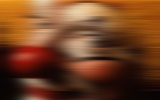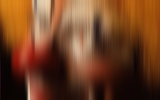There are two main ways to execute an op: 1. The type-safe way using a built-in method: ij.op().foo().bar(...)  This way tends to be nicer from type-safe languages like Java. 2. The dynamic way using the run method: ij.op().run("foo.bar", ...)  This way tends to be nicer from dynamically typed scripting languages. With the dynamic approach, passing the namespace is optional, so you can also write: ij.op().run("bar", ...)  If there are ops with the same name across multiple namespaces (e.g., math.and and logic.and), then passing the short name will consider the op signatures across all namespaces. You will see both syntaxes used throughout these tutorials. ## Generating Images¶ Images have to come from somewhere. You can use ij.io().open(...) to read one in from an external source such as files on disk, like we did with previous notebooks. But there are other ways, too. ### Creating an empty image¶ You can create an empty (zero-filled) image using the create.img op. In : ij.op().help("create.img")  Out: Available operations: (Img out) = net.imagej.ops.create.img.CreateImgFromImg( Img in) (Img out) = net.imagej.ops.create.img.CreateImgFromII( IterableInterval in) (Img out) = net.imagej.ops.create.img.CreateImgFromRAI( RandomAccessibleInterval in) (Img out) = net.imagej.ops.create.img.CreateImgFromDimsAndType( Dimensions in1, NativeType in2, ImgFactory factory?) (Img out) = net.imagej.ops.create.img.CreateImgFromInterval( Interval in) Here we will use the clown image as a reference image. In : def info(name, image) { imageType = image.firstElement().getClass().getSimpleName() name + " = " + image.dimension(0) + " x " + image.dimension(1) + " x " + image.dimension(2) + " : " + imageType + "\n" } // Create an empty image of the same size as an existing image. empty = ij.op().run("create.img", image) // Create an empty image based on another image, but of different type. import net.imglib2.type.logic.BitType bitType = ij.op().run("create.img", image, new BitType()) // Create an image from scratch. import net.imglib2.type.numeric.real.FloatType smallFloat = ij.op().run("create.img", [25, 17, 2], new FloatType()) info("Original ", image) + info("Empty one", empty) + info("Bit image", bitType) + info("Small float", smallFloat)  Out: Original = 160 x 100 x 3 : UnsignedByteType Empty one = 160 x 100 x 3 : UnsignedByteType Bit image = 160 x 100 x 3 : BitType Small float = 25 x 17 x 2 : FloatType  ### Copying an image¶ You can easily make a copy of an image using ops from the copy namespace. In : ij.op().help("copy.rai")  Out: Available operations: (RandomAccessibleInterval out?) = net.imagej.ops.copy.CopyRAI( RandomAccessibleInterval out?, RandomAccessibleInterval in) In : copy = ij.op().run("copy.rai", image)  Out:### Generating an image from a formula¶ The equation op allows you to generate an image from a formula in JavaScript syntax. Such images can be useful for testing without needing an external image source, or a long and bulky list of numbers. This Op will execute the equation on each pixel of the image. Within the equation the current location can be retrieved via the variable p[] (see example equation below). In : ij.op().help("equation")  Out: Available operations: (IterableInterval out) = net.imagej.ops.image.equation.DefaultCoordinatesEquation( IterableInterval out, UnaryFunctionOp in) (IterableInterval out?) = net.imagej.ops.image.equation.DefaultEquation( IterableInterval out?, String in) (IterableInterval out) = net.imagej.ops.image.equation.DefaultXYEquation( IterableInterval out, DoubleBinaryOperator in) In : import net.imglib2.type.numeric.integer.UnsignedByteType sinusoid = ij.op().run("create.img", [150, 100], new UnsignedByteType()) formula = "63 * (Math.cos(0.3*p) + Math.sin(0.3*p)) + 127" ij.op().image().equation(sinusoid, formula)  Out: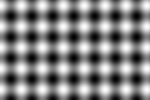### Wrapping an array as an image¶ There is no op yet to create an image by wrapping an existing array of values. For now, you can use ImgLib2 utility methods for that: In : // Here we have a gradient ramp in an array. w = 160; h = 96 byte[] pixels = new byte[w * h] for (y in 0..h-1) { for (x in 0..w-1) { pixels[y * w + x] = x + y } } // Wrap the array into an image. import net.imglib2.img.array.ArrayImgs ramp = ArrayImgs.unsignedBytes(pixels, w, h)  Out:## Computing Statistics¶ The stats package has routines for computing numerical statistics. Here is a rundown of many of them: In : sinusoid32 = ij.op().run("create.img", [150, 100]) formula = "63 * (Math.cos(0.3*p) + Math.sin(0.3*p)) + 127" ij.op().image().equation(sinusoid32, formula) ij.notebook().display([ "geometricMean": ij.op().stats().geometricMean(sinusoid32).toString(), "harmonicMean": ij.op().stats().harmonicMean(sinusoid32).toString(), "kurtosis": ij.op().stats().kurtosis(sinusoid32).toString(), "max": ij.op().stats().max(sinusoid32).toString(), "mean": ij.op().stats().mean(sinusoid32).toString(), "median": ij.op().stats().median(sinusoid32).toString(), "min": ij.op().stats().min(sinusoid32).toString(), "moment1AboutMean": ij.op().stats().moment1AboutMean(sinusoid32).toString(), "moment2AboutMean": ij.op().stats().moment2AboutMean(sinusoid32).toString(), "moment3AboutMean": ij.op().stats().moment3AboutMean(sinusoid32).toString(), "moment4AboutMean": ij.op().stats().moment4AboutMean(sinusoid32).toString(), "size": ij.op().stats().size(sinusoid32).toString(), "skewness": ij.op().stats().skewness(sinusoid32).toString(), "stdDev": ij.op().stats().stdDev(sinusoid32).toString(), "sum": ij.op().stats().sum(sinusoid32).toString(), "sumOfInverses": ij.op().stats().sumOfInverses(sinusoid32).toString(), "sumOfLogs": ij.op().stats().sumOfLogs(sinusoid32).toString(), "sumOfSquares": ij.op().stats().sumOfSquares(sinusoid32).toString(), "variance": ij.op().stats().variance(sinusoid32).toString() ])  Out:  geometricMean 106.864 harmonicMean 60.8145 kurtosis 2.25039 max 253 mean 130.356 median 129.34 min 1.29381 moment1AboutMean -2.87721e-13 moment2AboutMean 3982.16 moment3AboutMean -10222 moment4AboutMean 3.56905e+07 size 15000 skewness -0.0406737 stdDev 63.1064 sum 1.95534e+06 sumOfInverses 246.652 sumOfLogs 70073.4 sumOfSquares 3.14622e+08 variance 3982.42 ## Math on Image¶ In the math namespace, Ops provides traditional mathematical operations such as add, subtract, multiply and divide. These operations are overloaded in several ways: • Operate pixelwise between two images—e.g., math.add(image1, image2) when image1 and image2 have the same dimensions. • Operate between an image and a constant—e.g., math.add(image, 5) to add 5 to each sample of image. • Operate between two numerical values—e.g., math.add(3, 5) to compute the sum of 3 and 5. Some math ops are also already heavily optimized, since we used the math.add op as a testbed to validate that Ops could perform as well or better than ImageJ 1.x does. In : // Prepare a couple of equally sized images. import net.imglib2.type.numeric.real.FloatType image1 = ij.op().run("create.img", [160, 96], new FloatType()) image2 = ij.op().run("copy.rai", image1) // Gradient toward bottom right. ij.op().image().equation(image1, "p + p") minMax1 = ij.op().stats().minMax(image1) println("image1 range = (" + minMax1.getA() + ", " + minMax1.getB() + ")") // Sinusoid. ij.op().image().equation(image2, "64 * (Math.sin(0.1 * p) + Math.cos(0.1 * p)) + 128") minMax2 = ij.op().stats().minMax(image2) println("image2 range = (" + minMax2.getA() + ", " + minMax2.getB() + ")") ij.notebook().display([["image1":image1, "image2":image2]])  image1 range = (0.0, 254.0) image2 range = (0.020272091031074524, 255.97271728515625)  Out: image1image2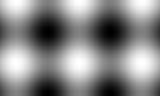Let's test math.add(image, number): In : addImage = image1 // Try also with image2! tile([ addImage, ij.op().run("math.add", ij.op().run("copy.rai", addImage), 60), ij.op().run("math.add", ij.op().run("copy.rai", addImage), 120), ij.op().run("math.add", ij.op().run("copy.rai", addImage), 180) ])  Out:Notice how we had to make a copy of the source image for each add(image, number) above? This is because right now, the best-matching math.add op is an inplace operation, modifying the source image. Ops is still young, and needs more fine tuning! In the meantime, watch out for details like this. Now we'll try math.add(image1, image2) and math.subtract(image1, image2): In : sum = ij.op().run("math.add", image1, image2) diff = ij.op().run("math.subtract", image1, image2) tile([sum, diff])  Out:Here is math.multiply(image1, image2): In : ij.op().run("math.multiply", image1, image2)  Out: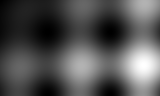And finally math.divide(image1, image2): In : ij.op().run("math.divide", image1, image2)  Out: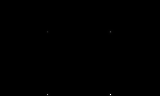## Evaluating expressions¶ ImageJ Ops offers a powerful expression evaluation op, built on SciJava's Parsington library: In : ij.op().help("eval")  Out: Available operations: (Object out) = net.imagej.ops.eval.DefaultEval( String in, Map vars?) Operators in the expression map to ops as follows: In : import net.imagej.ops.eval.OpEvaluator ij.notebook().display(new OpEvaluator().getOpMap().entrySet().stream().map{ entry -> ["Operator": entry.getKey().toString(), "Op name": entry.getValue()] }.collect())  Out: OperatorOp name ||logic.or >>>math.unsignedRightShift +math.add ==logic.equal &&logic.and >logic.greaterThan >=logic.greaterThanOrEqual <<math.leftShift !=logic.notEqual -math.negate +identity %math.remainder /math.divide &math.and |math.or -math.subtract >>math.rightShift ^math.power *math.multiply <logic.lessThan <=logic.lessThanOrEqual You can also call any op in an eval statement as a function, using familiar function syntax. The following is an example of the eval op being used to compute a Difference of Gaussians. Inputs within the formula may be accessed via the Map vars? argument of the eval function and the key of the map corresponds to the name of the variable that can be used in the formula. In : dogFormula = "gauss(image, [10, 10, 1]) - gauss(image, [5, 5, 1])" dog = ij.op().eval(dogFormula, ["image": image32])  Out:## Converting between image types¶ This tutorial illustrates ways to convert between pixel types. In : dogFormula = "gauss(image, [10, 10, 1]) - gauss(image, [5, 5, 1])" dog = ij.op().eval(dogFormula, ["image": image32])  Out:Did you notice in the previous cell that we computed the gauss on a float32 version of the image? If we do not do this, the result will be wrong: In : dogWrong = ij.op().eval(dogFormula, ["image": grayImage]) dogConverted = ij.op().convert().uint8(dog) ij.notebook().display([["dogWrong":dogWrong, "dogConverted":dogConverted]])  Out: dogWrongdogConvertedAs you can see, a DoG computed on the original uint8 version of the image looks essentially the same as the float32 DoG converted to uint8. The issue is that uint8 images have only 8 bits per channel, with values ranging from 0 to 255. So while the equation is dutifully evaluated, non-integer values are truncated, and then only the lowest 8 bits are retained. Using a richer image type like float32 avoids the problem. The float32 type uses 32-bit IEEE floating point numbers for its samples, which an approximate range of$-3.4 \times 10^{38}$to$3.4 \times 10^{38}$. This type requires four times as much memory as uint8, but math errors will accumulate much less severely in common cases.For those familiar with ImageJ 1.x, float32 corresponds to ImageJ 1.x's "32-bit" type in the Image → Type menu, and is typically what people choose when performing sequences of mathematical operations. ### Built-in image types¶ In ImageJ Ops, many image types are available: In : ij.op().ops().findAll{op -> op.startsWith("convert.big") || op.startsWith("convert.bit") || op.startsWith("convert.cfloat") || op.startsWith("convert.float") || op.startsWith("convert.int") || op.startsWith("convert.uint") }.collect{op -> op[8..-1]}  Out: [bit, cfloat32, cfloat64, float32, float64, int16, int32, int64, int8, uint12, uint128, uint16, uint2, uint32, uint4, uint64, uint8] In some circumstances, one of the above types is more appropriate than float32. For example, if we are squaring an image, we could use uint16, since the square of a number from 0 to 255 will always be in the range of 0 to 65535. It may sometimes make sense to use a high-precision integer type such as uint128 rather than a potentially lossy floating point type like float32. ### Methods of type conversion¶ Ops provides four built-in ways of converting image values across types: • convert.copy tranfers the values directly. This is the fastest method, but there is no bounds checking, so you may see overflow, or in some cases even end up with invalid sample values. • convert.clip is like copy but includes bounds checking. Values less than the minimum are clipped (also called clamped) to the minimum value, and values greater than the maximum are clipped to the maximum. • convert.scale multiplies sample values by a scale factor, such that the intensities fall into the same relative histogram over the whole target type:$[sourceTypeMin, sourceTypeMax] \to [destTypeMin, destTypeMax]$. E.g., converting from uint8 to uint16 linearly scales$[0, 255] \to [0, 65535]$. • convert.normalizeScale multiplies sample values by a scale factor, such that intensities are distributed across the full range of the target type:$[sourceDataMin, sourceDataMax] \to [destTypeMin, destTypeMax]$. E.g., converting a uint8 image with an actual minimum sample value of 16 and actual maximum sample value of 127 to uint16 linearly scales$[16, 127] \to [0, 65535]\$.

Below, we show how to convert images using these approaches.

In :
// Make a handy method for printing the image min/max.
printMinMax = { prefix, image ->
minMax = ij.op().stats().minMax(image)
println(prefix + ": min = " + minMax.getA() + ", max = " + minMax.getB())
}

// Create an image with an illustrative range of intensities.
import net.imglib2.type.numeric.integer.UnsignedShortType
imageToConvert = ij.op().run("create.img", [96, 64], new UnsignedShortType())
formula = "128 * (Math.cos(0.3*p) + Math.sin(0.3*p)) + 384"
ij.op().image().equation(imageToConvert, formula)
printMinMax("Original", imageToConvert)
imageToConvert

Original: min = 129, max = 640

Out: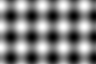Now we will call the various convert ops. Please be aware that these ops currently have architectural issues, which we plan to address in a future version of ImageJ Ops. The code below works, but is subject to change until the ImageJ Ops 1.0.0 release.

In :
import net.imagej.ops.Ops
import net.imagej.ops.special.computer.Computers
import net.imglib2.type.numeric.integer.UnsignedByteType

convertMethods = [
Ops.Convert.Copy.class,
Ops.Convert.Clip.class,
// HACK: convert.scale op is disabled here due to a bug in Ops.
//  Ops.Convert.Scale.class,
Ops.Convert.NormalizeScale.class
]

convertedImages = []
for (convertMethod in convertMethods) {
// Create a uint8 destination image for the conversion.
convertedImage = ij.op().run("create.img", imageToConvert, new UnsignedByteType())

// Look up the needed convert op for converting from source to destination.
inType = imageToConvert.firstElement() // Type from which we are converting.
outType = convertedImage.firstElement() // Type to which we are converting.
convertOp = Computers.unary(ij.op(), convertMethod, outType, inType)

// NB: Prepare the convert op to behave properly.
// The need for these calls is hacky, and will be fixed in a future version of Ops.
convertOp.checkInput(inType, outType)
convertOp.checkInput(imageToConvert)

// Run this convert op on every sample of our source image.
ij.op().run("map", convertedImage, imageToConvert, convertOp)
methodName = convertMethod.getField("NAME").get(null)
printMinMax(methodName, convertedImage)
}

tile(convertedImages)

convert.copy: min = 0, max = 255
convert.clip: min = 129, max = 255
convert.normalizeScale: min = 0, max = 255

Out: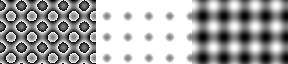The code above makes use of the map op to execute the appropriate convert op on each element of a collection.

## Filtering¶

This notebook demonstrates various image filtering operations. There are lots of image filtering operations available in the filter namespace:

In :
ij.op().ops().findAll{op ->
op.startsWith("filter.")
}.collect{op -> op[7..-1]}

Out:
[addNoise, addPoissonNoise, allPartialDerivatives, bilateral, convolve, correlate, createFFTOutput, derivativeGauss, dog, fft, fftSize, frangiVesselness, gauss, hessian, ifft, linearFilter, max, mean, median, min, padFFTInput, padInput, padShiftFFTKernel, paddingIntervalCentered, paddingIntervalOrigin, partialDerivative, sigma, sobel, tubeness, variance]

Here is a demonstration of some of them:

In :
ij.op().help("filter.addPoissonNoise")

Out:
Available operations:
(RealType out) =
RealType out,
RealType in,
long seed?)
(IterableInterval out) =
IterableInterval out,
IterableInterval in)
In :
imageToFilter = image32

// We will use a 3x3 diamond as our neighborhood here.
import net.imglib2.algorithm.neighborhood.DiamondShape

// Gaussian blur.

// Median filter.
median = ij.op().run("create.img", imageToFilter)
ij.op().filter().median(median, imageToFilter, shape)

// Min filter.
min = ij.op().run("create.img", imageToFilter)
ij.op().filter().min(min, imageToFilter, shape)

// Max filter.
max = ij.op().run("create.img", imageToFilter)
ij.op().filter().max(max, imageToFilter, shape)

// Sobel filter.
sobel = ij.op().filter().sobel(imageToFilter)

// Display the results side by side.
ij.notebook().display([